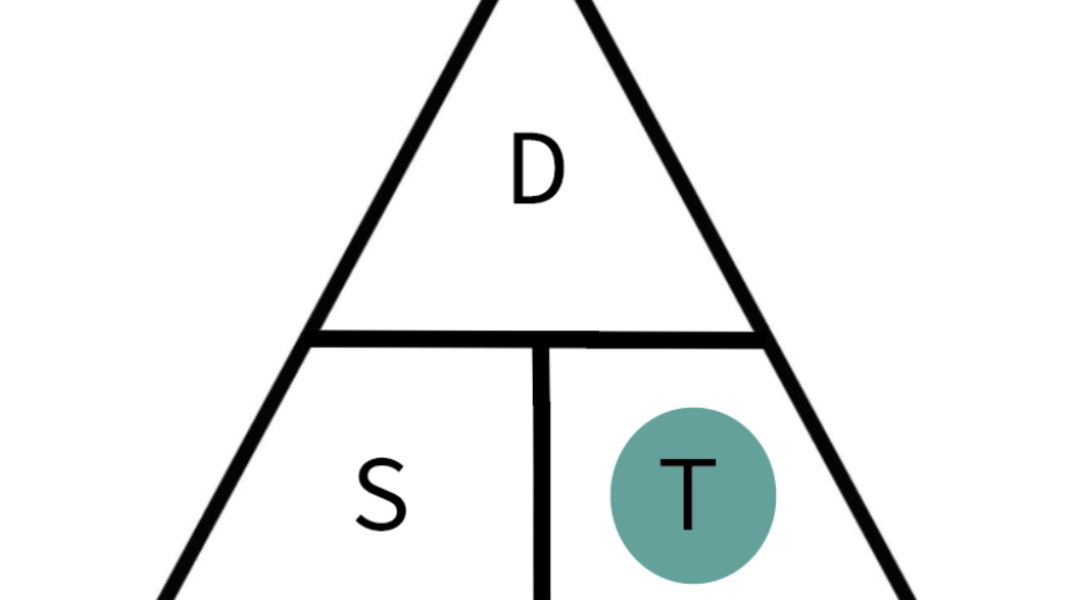Working out speed, distance and time is an important part of many roles, including those in the armed forces or transport industries.

If you are applying for a role in these industries, you may be tested on this as part of your recruitment process. The questions will allow your employer to test your applied mathematical abilities.

## How to calculate speed, distance & time: the formula triangle you’ll need?

To work out speed, divide the distance of the journey by the time it took to travel, so speed = distance divided by time. To calculate time, divide the distance by speed. To get the distance, multiply the speed by time.

You may see these equations simplified as s=d/t, where s is speed, d is distance, and t is time.This formula can be arranged into the triangle above. In the triangle, speed and time form the base, as they are what is multiplied together to work out the distance.

The triangle is an easy way to remember the formula and can save you time when working out your exam questions. The triangle will help you remember the three formulas:

• The formula of speed is Speed = Distance ÷ Time
• The formula of time is Time = Distance ÷ Speed
• The formula of distance is Distance = Speed x Time

The triangle shows you what calculation you should use. As distance is at the top of the triangle, to work it out, you need to multiply speed with time.

As speed and time are at the bottom of the triangle, you need to divide this number from the figure for distance, to work out the correct answer.

When beginning to study for your practice test, make sure you write out the triangle on your paper. This will help you to memorize it.

## How to calculate speedTo calculate speed, you need to divide the distance by the time. You can work this out by using the triangle. If you cover up speed, you are left with distance over time.

Here is an example:

If a driver has travelled 180 miles and it took them 3 hours to make that distance, then to work out their speed you would take:

180 miles / 3 hours -\> 180 / 3 = 60

So the driver’s speed would be 60mph.

## How to calculate distanceTo work out the distance travelled, you will need to multiply speed and time.

Here is an example:

If a driver travelled at 100mph for 4 hours, then to work out the distance you will need to multiply the speed with the time.

100mph x 4 hours -\> 100 x 4 = 400

The distance is 400 miles.

## How to calculate the timeTo work out the time that the journey took, you will need to know the speed of the journey and the distance that was travelled.

Here is an example:

If the driver travelled 50 miles at 5mph, then to work out the time taken you would divide:

50miles / 5 miles per hour -\> 50 / 5 = 10

The time taken to travel this distance is 10 miles per hour.

## Three example speed/distance/time questions

1. Andy drives his lorry for 400 miles, which takes him 8 hours. Harry drives 200 miles, which takes him 4.5 hours. Who is travelling faster?

Answer: Andy is driving at 50mph, and Harry is driving at 44.44mph. So, Andy is driving faster.

1. Tessa runs a 5km race with her running club every Saturday. She runs this in 40 minutes. If she maintains the same speed, how long would it take her to run the 8km race?

Answer: Her speed is 7.5kph. If she runs 10km at 7.5kph, to work out the time, you need to divide 8 by 7.5, which equals 1.066. If we convert this into hours and minutes, it will take her 1 hour and 4 minutes to run 8km.

1. Hannah goes on a cycling trip. In the first half of the journey, she travels at 10mph for 2 hours. In the second half, she travels at 20mph for 90 minutes. How far does she travel in total?

Answer: In the first half of her journey she travels 20 miles (10 x 2 = 20). In the second half, she travels 30 miles, (20 x 1.5 = 30). 20+30 =50, so she travels 50 miles in total.

## Methods to get better at these questions

To improve your skill in answering speed distance time questions, there are two main things you can do.

First, make sure that you are familiar with the formula triangle. The key to answering these questions is knowing the formula inside out, so that you always know what equation to use, whether the exam question is asking you to work out speed or distance.

By making sure you have memorized the formula in all its variations, you will save yourself time when answering questions.

Secondly, you should focus on improving your general mathematical skills. You may get a compound question, which asks you to use the formula in conjunction with other maths skills to work out the answer to the problem.

You can try a few maths practice tests, such as these, which will help you to practise your basic numeracy skills.